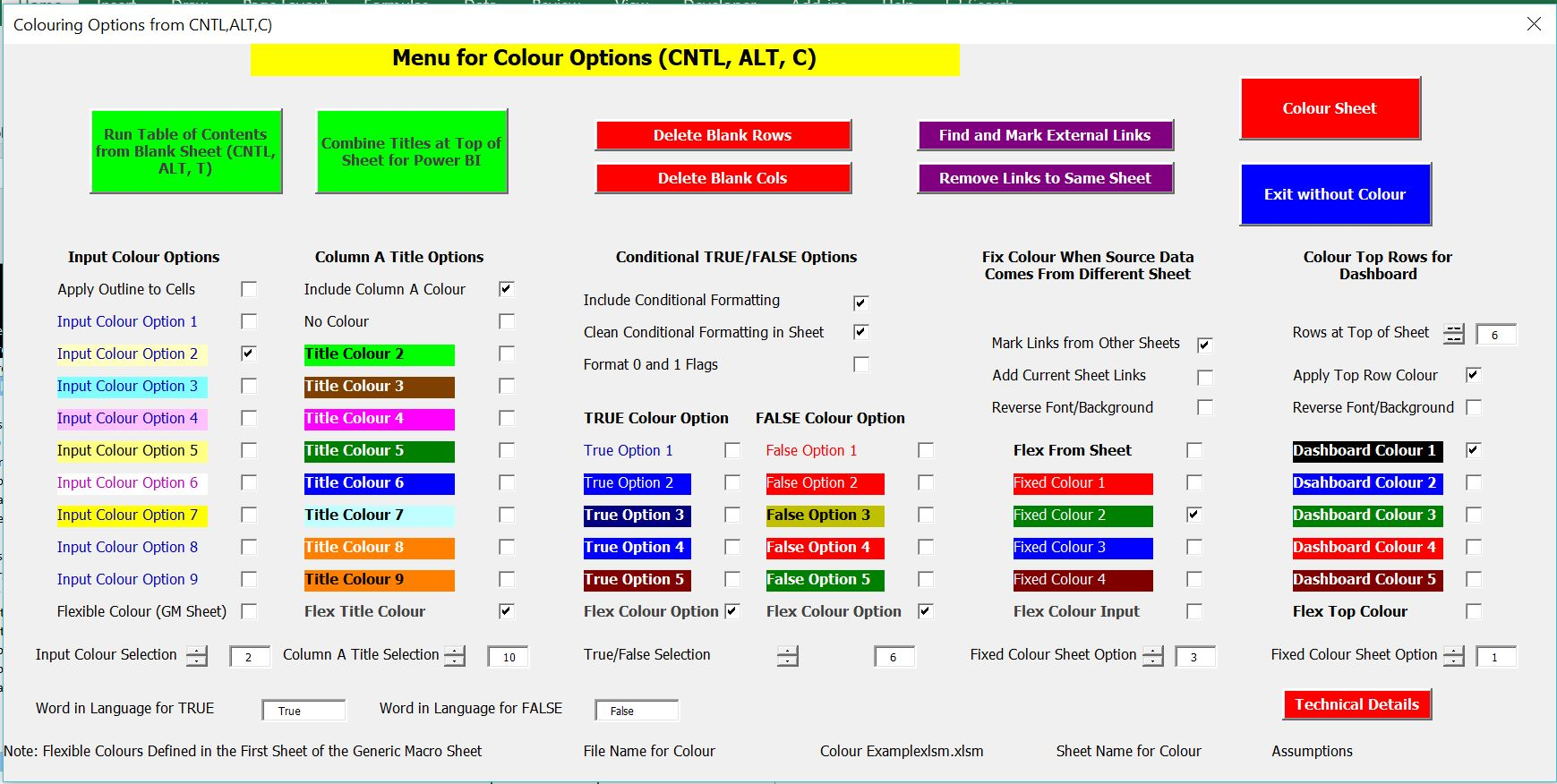This page shows how to create a macro that finds cells in your workbook that refer to an external file. Most people hate the idea of using an external file but sometimes it can be necessary.  Through using a macro, you can point out any cells that are referring to another sheet in all of the formulas in that use the current sheet name of your workbook. The method used is to get the sheet name with a function and then use the instring function to replace the sheet name with a function. The VBA code for running this program is shown in this page.

## Mechanics of Running the External Link Macro if you Don’t Care About the VBA

If you refer to another workbook in a formula, the current sheet name will appear in formula. You can see all of the links with the short-cut:

Alt, E, K

The results of this short-cut is shown in the screenshot below. Note that if there are no external links, the short-cut, ALT, E, K does not work.To mark the sheet links you can use the Generic Macros excel file. One of the buttons on the form that appears after pressing CNTL, ALT, C will scan a sheet and colour cells that have external links. The purple button that allows you to do this from pressing CNTL, ALT, C is shown in the screenshot below.After you run the macro, the external link will be coloured as illustrated in the screenshot below. Note that you could modify the macro to use a different format and/or scan all of the sheets.

## VBA Code for Marking External Links

The VBA code below can be copied into your sheet or your personal workbook if you want to use it.  Note that ost of the code is simply about formatting. The code demonstrates how you can work through each row and column in an excel sheet.  The macro shown below works through cells in a sheet and then once it finds the [ it formats the cell.

.

```'-----------------------------------------------------------------------------------------------------------
' This program find the external links and colours them
'-----------------------------------------------------------------------------------------------------------

x_find_rows
x_find_cols

max_col = WorksheetFunction.Min(max_col, 50) ' Limit the cols
max_row = WorksheetFunction.Min(max_row, 800) ' Limit the rows

For row = 1 To max_row
For col = 1 To max_col
char_formula = Cells(row, col).Formula
formula_length = Len(char_formula)

For cell_char = 1 To formula_length
cell_item = ""
cell_item = Mid(Cells(row, col).Formula, cell_char, 1)

If cell_item = "[" Then

' MsgBox " found link " & char_length

Cells(row, col).Select
Selection.Font.Bold = True
Selection.Font.Italic = True

GoTo format_cell:
resume_analysis:

End If
Next cell_char

Application.StatusBar = " Row " & row & " Column " & col

Next col
Next row

Range("A1").Activate

Application.StatusBar = False

Exit Sub

'
format_cell:
Cells(row, col).Select
Selection.Font.Bold = True
Selection.Font.Italic = True
With Selection.Font
.Color = -65536
End With
With Selection.Interior
.Pattern = xlSolid
.PatternColorIndex = xlAutomatic
.ThemeColor = xlThemeColorAccent6
End With
Selection.Borders(xlDiagonalDown).LineStyle = xlNone
Selection.Borders(xlDiagonalUp).LineStyle = xlNone
With Selection.Borders(xlEdgeLeft)
.LineStyle = xlContinuous
.ColorIndex = 0
.Weight = xlMedium
End With
With Selection.Borders(xlEdgeTop)
.LineStyle = xlContinuous
.ColorIndex = 0
.Weight = xlMedium
End With
With Selection.Borders(xlEdgeBottom)
.LineStyle = xlContinuous
.ColorIndex = 0
.Weight = xlMedium
End With
With Selection.Borders(xlEdgeRight)
.LineStyle = xlContinuous
.ColorIndex = 0
.Weight = xlMedium
End With
Selection.Borders(xlInsideVertical).LineStyle = xlNone
Selection.Borders(xlInsideHorizontal).LineStyle = xlNone

GoTo resume_analysis:

End Sub

.

```